Next: 2.1.3 Penetration Depth for dx-y-Wave Up: 2.1 The Magnetic Penetration Previous: 2.1.1 The London Penetration

## 2.1.2 The BCS Penetration Depth

Consider the BCS ground state for a conventional superconductor with a cylindrical Fermi surface, such that the axis of the Fermi cylinder is parallel to the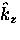-axis. A cross-section of the Fermi cylinder in the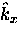-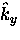plane is shown in Fig 2.2. In the superconducting ground state, the electrons near the Fermi surface are paired through a virtual phonon-induced attractive interaction (i.e. Cooper pairs). The BCS ground state differs from the normal state in that some of the states just above the normal Fermi surface are occupied, while some just below are unoccupied. The attractive interaction between paired electrons lowers the total energy relative to the normal ground state of unpaired electrons. The energy reduction is maximized if the two electrons making up a pair (i) have equal and opposite momenta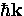and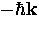, so that the total center-of-mass momentum of a Cooper pair is zero and (ii) if they have opposite spins (i.e. in a spin-singlet state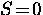). The two electrons which make up a Cooper pair are continuously scattered between states of equal and opposite momentum by virtual phonons. However, the scattering pairs interfere with each other so that the superconducting state is a highly correlated many-body state. The BCS theory calculates the superconducting ground state using an explicit N-particle wavefunction with all of these correlations built in. The wavefunctions corresponding to the Cooper pairs in the original BCS theory were assumed to have orbital angular momentum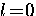. Thus, in a conventional superconductor the pairing is considered to be s-wave, spin singlet. At a given instant of time, a Cooper pair is in a state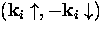,where the wavefunction describing the pair consists of all states i'' occupied by the pair during its lifetime. It should be noted that the original BCS theory can be extended to explain other systems. For instance, the BCS theory can be generalized to describe the superfluid state of 3He. The 3He atoms form p-wave (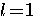) spin-triplet (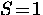)pairs. In this case, the 3He atoms are held together by magnetic interactions, rather than phonons.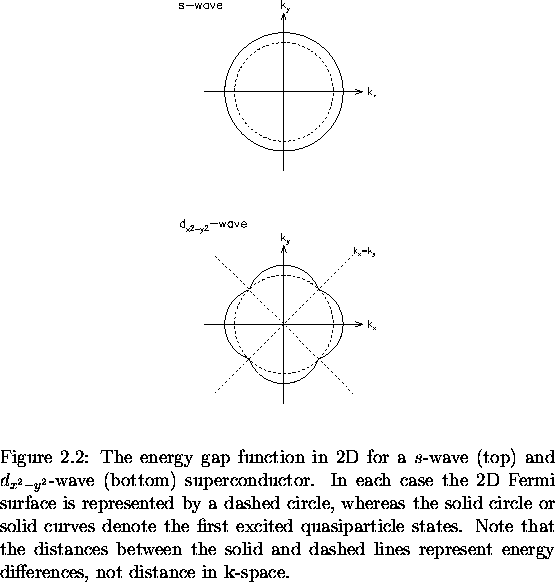One of the important features emerging from the BCS theory, is the existence of a temperature-dependent energy gap function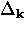at the Fermi surface. For an energy gap with s-wave symmetry,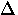is independent of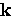. The energy gapis the minimum energy required to create a single electron (hole) excitation from the superconducting ground state, so that the binding energy of a Cooper pair is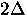.Many conventional superconductors are in the weak-coupling limit in which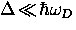, where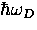is the Debye energy for phonons in the lattice. In the weak-coupling limit at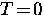, the uniform energy gap is simply proportional to Tc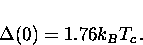(22)
At arbitrary temperature the energy gap is given by(23)
where f is a universal function of the ratio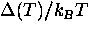.Near Tc this yields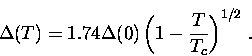(24)
As the temperature is raised above, an increasing number of electrons are thermally excited across the energy gap into single quasiparticle states. The minimum energy to create an excitation is, which is the binding energy of a Cooper pair. A single quasiparticle excitation of momentumhas energy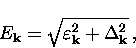(25)
whereis the quasiparticle energy in the absence of a gap measured relative to the Fermi surface.

The penetration depth in BCS theory is determined by first calculating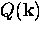and then substituting the result into Eq. (2.5). The expression for the BCS kernel is nonlocal, and therefore more general than the London kernel. Since the expression forand hence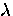turns out to be nontrivial, only the BCS result in the low-temperature limit is presented here. In particular, for a clean superconductor in which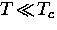,the number of quasiparticles excited to energy states above the gap is exponentially activated such that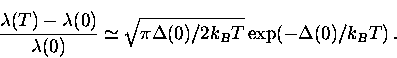(26)Next: 2.1.3 Penetration Depth for dx-y-Wave Up: 2.1 The Magnetic Penetration Previous: 2.1.1 The London Penetration